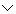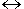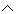# EquivalenceExample 1:

 Given: ~pq If I don't study, then I fail. pq I study or I fail. Problem: Determine the truth values of the given statements.

Solution:

 p q ~p ~pq pq T T F T T T F F T T F T T T T F F T F F

In the truth table above, the last two columns have the same exact truth values!  Therefore, the statement ~pq is logically equivalent to the statement pq.

Definition: When two statements have the same exact truth values, they are said to be logically equivalent.

Example 2: Construct a truth table for each statement below. Then determine which two are logically equivalent.

1. ~qp

2. ~(pq)

3. pq

 p q ~q ~qp T T F T T F T T F T F T F F T F
 p q pq ~(pq) T T T F T F F T F T T F F F T F
 p q pq T T T T F T F T T F F F

The truth tables above show that ~qp is logically equivalent to pq, since these statements have the same exact truth values. In Example 3, we will place the truth values of these two equivalent statements side by side in the same truth table. We will then examine the biconditional of these statements.

Example 3: Construct a truth table for (~qp)(pq)

 p q ~q ~qp pq (~qp)(pq) T T F T T T T F T T T T F T F T T T F F T F F T

The biconditional (~qp)( pq) is a tautology. This is no coincidence:  It turns out that any two equivalent statements will yield a tautology when placed in the biconditional.

Definition: The biconditional of two equivalent statements is a tautology.

In the next example, we will place the two equivalent statements from Example 1 in the biconditional.

Example 4:

 Given: ~pq If I don't study, then I fail. pq I study or I fail. Problem: Is (~pq)(pq) a tautology?

Solution:

 p q ~p ~pq pq (~pq)(pq) T T F T T T T F F T T T F T T T T T F F T F F T

The truth values of biconditional (~pq)(pq) are {T, T, T, T}. Therefore, (~pq)(pq) is a tautology. Once again, we see that the biconditional of two equivalent statements is a tautology.Summary:

When two statements have the same exact truth values, they are said to be logically equivalent. The biconditional of two equivalent statements is a tautology.

Exercises

 1. What are the truth values of the following statement? (p~q)~p {T, T, T, F}{T, F, T, T}{F, T, T, T}None of the above. RESULTS BOX:
 2. Which of the following statements is logically equivalent to the statement given in Exercise 1? qp~p(p~q)pqNone of the above. RESULTS BOX:
 3. Which of the following statements is logically equivalent to q(pq)? qp~p(p~q)pqNone of the above. RESULTS BOX:
 4. Which of the following statements is logically equivalent to a(ab)? ab(ab)b(ab)bNone of the above. RESULTS BOX:
5.
 Given: Statement x is logically equivalent to statement y. Problem: Which of the following is true?

x if and only if y
xy is a tautology
x iff y
All of the above.

RESULTS BOX:

 Lessons on Symbolic Logic Negation Conjunction Disjunction Conditional Compound Biconditional Tautologies Equivalence Practice Exercises Challenge Exercises Solutions